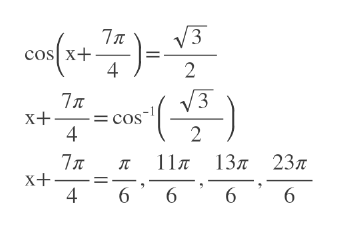# solve the equation 2cos(x+   7π/4)=√3 giving the exact solution which lie in [0,2π)

Question

solve the equation 2cos(x+   7π/4)=√3 giving the exact solution which lie in [0,2π)

check_circleExpert Solution
Step 1

Divide by 2.

Step 2

Take cos inverse. For cos^-1(sqrt(3)/2) too...help_outlineImage Transcriptionclose3 7л cos X+ 4 2 3 7л x+ cos 4 2 л 11л 13л 23л 6 7 x X+ 4 6 6 6 fullscreen

### Want to see the full answer?

See Solution

#### Want to see this answer and more?

Solutions are written by subject experts who are available 24/7. Questions are typically answered within 1 hour*

See Solution
*Response times may vary by subject and question
Tagged in

### Other